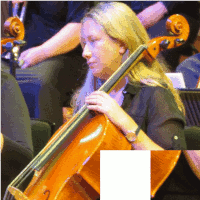# Sliding Blocks PuzzleI thought it would be fun to create a sliding-block puzzle on-screen. However I lost the code when I accidentally copied an 8G card image over the firtst 8G of my hard drive! This is therefore a re-creation, and bits are yet to add ( see the remarks in the code). I think an AI autosolver is beyond me however!

Code at present requires a 400x400 bitmap image called 'cellist.bmp', but fileselector will be added soon.

To be continued.....

```

'   ********************************************************************
'   **                                                                **
'   **    SlidingBlocks6a1.bas  tenochtitlanuk   17 Jan 2020          **
'   **                                                                **
'   ********************************************************************

'   To-dos
'       make the move conditional on whether adjacent block chosen and blank    DONE
'       get the graphics to work correctly- array seems to work & update        DONE

'       add option to choose image, including one showing numbers
'       test if all are in 'correct' places and announce number of attempted moves entered

'       add routine to display moves attempted
'       add routine to detect completion.
'       add routine to save moves so can reverse/rewind to start.....

nomainwin

global blankX, blankY, clicks

WindowWidth  = 412
WindowHeight = 440
UpperLeftX   = 800
UpperLeftY   =  20

open "Sliding blocks puzzle" for graphics_nsb as #wg

#wg "trapclose quit"

#wg "when leftButtonUp moveIfPossible"

#wg "drawbmp scr 1 1 ; flush"

dim puzzle\$( 5, 5)

i =1

for y =1 to 4   '   save tile00 to tile15
for x =1 to 4
#wg "getbmp tile" +right\$( "00" +str\$( i), 2); " "; ( x -1) *100; " "; ( y -1) *100; " 100 100"
'bmpsave "tile", "tile_" +right\$( "00" +str\$( i), 2)
puzzle\$( x, y) ="tile" +right\$( "00" +str\$( x +( y -1) *4), 2)
i =i +1
next x
next y

#wg "cls"   '   and now tile16, all white blank one
#wg "getbmp tile16 300 300 100 100"
#wg "cls"

blankX =4
blankY =4
clicks =0

'   fill the puzzle area systematically
'for i =1 to 15  '   tile16 is the white one...
'    #wg "drawbmp tile" +right\$( "00" +str\$( i), 2); " "; 100 *( ( i -1) mod 4); " "; 100 *( int( ( i -1) /4))
'next i
'        . .  . . . OR fill at random
posn\$       =" 01 02 03 04 05 06 07 08 09 10 11 12 13 14 15 16"
scrambled\$  =""
do
R           =int( len( posn\$) /3 *rnd( 1))              '   ie 0 ... 15
scrambled\$  =scrambled\$ +mid\$( posn\$, 1 +3 *R, 3)       '   3 chars   starting at 1, 4, 7, ..
lPart\$      =left\$( posn\$, 3 *R)                        '   all chars up to & inc 0, 3, 6, ....
rPart\$      =mid\$(  posn\$, 3 *R +4)                     '   all chars after & inc 4, 7,10, ....
posn\$       =lPart\$ +rPart\$
print scrambled\$

scan
loop until len( posn\$) =0

for i =0 to 15
x                       =( i mod 4)
y                       =int( i /4)
puzzle\$( x +1, y +1)    ="tile" +mid\$( scrambled\$, 3 *i +2, 2)
XX                      =val( mid\$( puzzle\$( x +1, y +1), 5, 1))
YY                      =val( mid\$( puzzle\$( x +1, y +1), 6, 1))
print "x+1, y+1 "; x+1; " "; y+1, "XX, YY "; XX; " "; YY; " tile = "; puzzle\$( x +1, y +1)
#wg "drawbmp "; puzzle\$( x+1, y+1); " "; 100 *x; " "; 100 *y
if str\$( XX) ="1" and str\$( YY) ="6" then blankX =x +1: blankY =y +1: print "Blank tile @ "; blankX; "; "; blankY
next i

print ""
for y =1 to 4
for x =1 to 4
print puzzle\$( x, y),
next x
print ""
next y

wait

sub quit h\$
close #wg
end
end sub

sub moveIfPossible h\$, x, y
'scan
if clicks =0 then
for y =1 to 4
for x =1 to 4
print puzzle\$( x, y),
next x
print ""
next y
end if

print ""

clicks                      =clicks +1

tileX                       =int( MouseX /100) +1   '   1 to 4 in x and y
tileY                       =int( MouseY /100) +1

if ( tileX =4) and ( tileY =4) and abs( tileX -blankX) =1 and ( tileY -blankY) =1 then notice "Home!!": goto [kk]
if ( tileX =blankX) and ( tileY =blankY)             then notice "You clicked space!": goto [skip]
if abs( tileX -blankX) >1 or abs( tileY -blankY) >1  then notice "Too far away!":      goto [skip]
if abs( tileX -blankX) =1 and abs( tileY -blankY) =1 then notice "Diagonal!":          goto [skip]
[kk]
print " Swap "; tileX; " ";  tileY; " "; puzzle\$(  tileX,  tileY); " with ";_
blankX; " "; blankY; " "; puzzle\$( blankX, blankY)

ch                          =(  tileX) +4 *(  tileY -1)
bl                          =( blankX) +4 *( blankY -1)

#wg   "drawbmp "; puzzle\$( blankX, blankY); " "; (  tileX -1) *100; " "; (  tileY -1) *100
#wg   "drawbmp "; puzzle\$(  tileX,  tileY); " "; ( blankX -1) *100; " "; ( blankY -1) *100

temp\$                       =puzzle\$( blankX, blankY)
puzzle\$( blankX, blankY)    =puzzle\$(  tileX,  tileY)
puzzle\$(  tileX,  tileY)    =temp\$

blankX                      =tileX
blankY                      =tileY

for y =1 to 4
for x =1 to 4
print puzzle\$( x, y),
next x
print ""
next y

print " Blank at "; blankX; ", "; blankY
print ""

#wg "getbmp all 0 0 399 399"
bmpsave "all", "all/all_" +right\$( "000" +str\$( clicks), 3) +".bmp"

[skip]
end sub

end

```